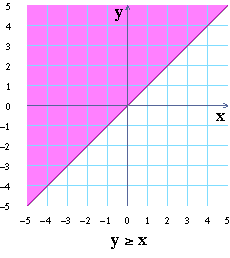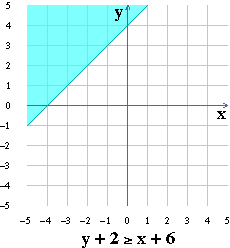# Equivalent Inequalities

Adding or multiplying by a nonzero constant on both sides of an equation doesn’t affect the equality. Does the same fact hold for inequalities? In this lesson you will study this question for linear inequalities.

Below are the graphs of \$y≥x\$ and \$y+2≥x+6\$.On the grid to the left, you are looking at the graphs of both of these inequalities on the same grid. The dark blue region is the overlapping region of the two inequalities. The pink region is the rest of the graph of \$y≥x\$.

 Use the slider to set \$a\$ to 0.5 in the inequality \$y+2≥x+a\$. The light blue region is that part of the graph of \$y+2≥x+0.5\$ that doesn’t overlap with the graph of \$y≥x\$. Find the coordinates of the red dot in the light blue region.
 Use the slider to find the value of \$a\$ (for \$y+2≥x+a\$) that makes the inequalities equivalent. This means that the graphs of the two inequalities should overlap each other completely, making the entire shaded region dark blue.
 Click to see the next example. Use the slider to find \$a\$ so that \$y
 Click . Use the slider to find \$a\$ so that \$y>x\$ is equivalent to \$y-3>x+a\$.
 Based on your answers to the last 3 questions, what value of \$a\$ makes \$y≤x\$ equivalent to \$y+7≤x+a\$?

Here are the graphs of \$y≥x\$ and \$y+a≥x+a\$. Remember that two inequalities are equivalent if they overlap completely (the shaded region is dark blue).

 Use the slider to change \$a\$. The inequalities you are looking at are written below the grid. Does adding the same constant to both sides of an inequality change the graph of the inequality?

## Multiplying by a constant

So far you have seen that adding the same constant to both sides of an inequality doesn’t change the graph of the inequality. Now, let’s look at multiplying both sides of an inequality by a constant.

 Use the slider to find the value of \$a\$ that makes the inequalities \$y≥x\$ and \$2y≥ax\$ equivalent.
 Click . Find \$a\$ so that \$y>x\$ is equivalent to \$7y>ax\$.
 Click . Find \$a\$ so that \$y≤x\$ is equivalent to \$3y≤ax\$.
 Click . Find \$a\$ so that \$y
 Based on your answers to the last 4 questions, what value of \$a\$ makes \$y≥x\$ equivalent to \$12y≥ax\$?

Use the slider to change \$a\$ and try to find a value for \$a\$ that makes the inequality \$y≥x\$ equivalent to \$-2y≥ax\$.

 In multByPos you could make the shaded regions overlap completely by making \$a\$ the same as the coefficient of \$y\$ (the number multiplying \$y\$). What is the coefficient of \$y\$ in this example (\$-2y≥ax\$)?
 You might think that if \$a=-2\$, the graphs will match. Slide \$a\$ to \$-2\$. Do the shaded regions overlap completely? (That is, are they the same?)
 Is the coefficient of \$y\$ positive or negative?
 In multByPos, was the coefficient of \$y\$ always positive, always negative, or neither?

In multByNeg, you saw that if you multiply both sides of an inequality by a negative number, you change the graph of the inequality. The question we are trying to answer is: "How can you multiply an inequality by a negative number and get an equivalent inequality?"

 Use the slider to find \$a\$ so that \$y≥x\$ is equivalent to \$-2y≤ax\$.

In multByNeg, you weren’t able to find any \$a\$ value that would make the inequalities equivalent.

 What is the inequality you are looking at in this example?
 What was the inequality you were looking at in multByNeg?

If one side of an inequality is multiplied by a negative number, to find an equivalent inequality the “direction” of the inequality has to change (for example, \$≥\$ has to change to \$≤\$).

 Find \$a\$ so that \$y>x\$ is equivalent to \$-3y
 Click . Use the graph and slider to fill in the blanks to the right with an inequality that is equivalent to \$y≤x\$.
 Based on your answers to the previous 2 questions, fill in the blanks to the right with an inequality that is equivalent to \$y>x\$.

You are looking at the graphs of \$y≥x\$ and \$ay≥ax\$. Remember that two inequalities are equivalent if their graphs overlap completely (the shaded region is dark blue).

 Use the slider to change \$a\$ between 0.1 and 1000. The inequalities you are looking at are written below the grid. Does multiplying by a positive constant on both sides of an inequality change the graph of the inequality?
 Click . Use the slider to change the value of \$a\$, and watch what happens to the graph of \$ay > ax\$. Does the graph change as you slide the slider from 10 to 1?
 Does it change as you slide the slider from 1 to \$-1\$?
 Does it change as you slide the slider from \$-1\$ to \$-10\$?
 Does multiplying by a negative constant on both sides on an inequality change the graph of the inequality?